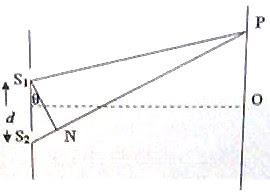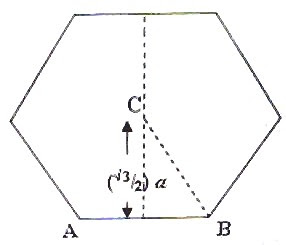## Sunday, September 28, 2008

### IIT-JEE 2008 Question (MCQ) on Young’s Double Slit

Today we will discuss two questions on Young’s double slit. The following multiple correct answers type question appeared in IIT-JEE 2008 question paper:

In a Young’s double slit experiment, the separation between the two slits is d and the wave length of the light is λ. The intensity of light falling on slit 1 is four times the intensity of light falling on slit 2. Choose the correct choice(s).

(A) If d = λ, the screen will contain only one maximum.

(B) If λ < d < 2λ, at least one more maximum (besides the central maximum) will be observed on the screen.

(C) If the intensity of light falling on slit 1 is reduced so that it becomes equal to that of slit 2, the intensities of the observed dark and bright fringes will increase.

(D) If the intensity of light falling on slit 2 is reduced so that it becomes equal to that of slit 1, the intensities of the observed dark and bright fringes will increaseThe path difference [S2N = (S2P S1P) in fig.] between the interfering beams is d sinθ. Therefore for obtaining a maximum at the point P on the screen the condition to be satisfied is

dsinθ =

When d = λ, sinθ = n

Since sinθ ≤ 1, n ≤ 1

The possible values of n are 0 and 1. But the maximum corresponding to n = 1 cannot be observed since θ cannot be 90º. Therefore, the central maximum of order zero corresponding to zero path difference (n = 0) alone can be observed. Option A is therefore correct

If λ < d < 2λ, the limiting values of d are λ and 2λ. In addition to the usual central maximum, fringes of order up to n satisfying the equation, dsinθ = will be obtained, where d < 2λ.

If d = 2λ, we have 2λ = nλ/sinθ so that n = 2 sinθ.

Since sinθ should be less than 1 for observing the fringe, the maximum value of n must be 1. Therefore, the central maximum and the maximum of order n = 1 will be observed. Option B too is therefore correct

Options C and D are obviously incorrect since the intensity of the dark fringes will become zero when the light beams passing through the slits are of equal intensity.

Now consider another question on Young’s double slit:

Suppose the wave length λ and the double slit separation d in a Young’s double slit experiment are such that the 6th dark fringe is obtained at point P shown in the above figure. The path difference (S2P – S1P) will be

(a) 5 λ

(b) 5 λ/2

(c) 6 λ

(d) 3 λ

(e) 11 λ/2

The dark fringe of order 1 (1st dark fringe) is formed when the path difference is λ/2. The dark fringe of order 2 is formed when the path difference is 3λ/2 (and not 2λ/2) and the dark fringe of order 3 is formed when the path difference is 5λ/2 (and not 3λ/2). Generally, the dark fringe of order n is formed when the path difference is (2n 1)λ/2. The 6th dark fringe is therefore formed when the path difference is 11λ/2.

By clicking on the label ‘wave optics’ below this post, you can access all related posts on this site.

You will find useful posts on wave optics here at apphysicsresources.

## Monday, September 22, 2008

### Moment of inertia - Two Multiple Choice Questions

The value of a man resides in what he gives and not in what he is capable of receiving

– Albert Einstein

You will find many questions involving moment of inertia on this site and you can access all of them by clicking on the label ‘MOMENT OF INERTIA’ below this post. I give you two more questions here, the first being the one which appeared in the AIEEE 2008 question paper. You will have to apply the parallel axis theorem to answer these questions.

(1) Consider a uniform square plate of side ‘a’ and mass ‘m’. The moment of inertia of this plate about an axis perpendicular to its plane and passing through one of its corners is

(1) (5/6) ma2

(2) (1/12) ma2(3) (7/12) ma2

(4) (2/3) ma2

The moment of inertia (ICM) of the square plate about an axis through its centre of mass (CM) passing perpendicular to its plane is given by

ICM = m(a2 + a2)/12 = ma2/6.

[Note that generally for a bar of rectangular cross section, the moment of inertia about an axis passing through the centre, perpendicular to its length is m(l2 + b2)12 where l is the length and b is the breadth].

The moment of inertia (I) about a parallel axis through one corner O is given by

I = ICM + m(a/√2)2 since the corner is at a distance a/√2 from the centre of mass (fig).

Thus I = ma2/6 + ma2/2 = (2/3) ma2

(2) A thin uniform rod of mass 6m is bent to form a regular hexagon of side a. What is the moment of inertia of the hexagon about an axis passing through its centre and perpendicular to its plane?(a) 5 ma2

(b) 5 ma2/6

(c) 5 ma2/3

(d) 6 ma2

(e) 6 ma2/5

Consider one side (such as AB) of the hexagon. Since its mass is m and its length is a, its moment of inertia about an axis through its centre and perpendicular to its length is given by

ICM = ma2/12.

The moment of inertia of this side about a parallel axis through the centre C of the hexagon is given by

I = ICM + m[(√3/2)a] 2 since the side is at a distance (√3/2)a from the centre of the hexagon (fig).

Thus I = ma2/12 + 3ma2/4 = 5 ma2/6.

Since there are six sides, the total moment of inertia of the hexagon is 6×5 ma2/6 = 5 ma2.

## Friday, September 12, 2008

### Resistive Networks- AIPMT 2008 Questions

The following questions on resistive networks appeared in All India Pre-Medical/Pre-Dental Entrance Examination 2008 question paper:

(1) In the circuit shown, the current through the 4 Ω resistor is 1 amp when the points P and M are connected to a d.c. voltage source. The potential difference between the points M and N is(1) 1.0 volt

(2) 0.5 volt

(2) 3.2 volt

(2) 1.5 volt

Since the current through the 4 Ω resistor is 1 ampere, the potential difference between the points P and M is 4 volt. The 1 Ω resistor and the two parallel resistors (each of value 0.5 Ω) make the total resistance in the branch PNM equal to 1.25 Ω. The potential drop across the 1 Ω resistor is therefore equal to 4×1/1.25 = 3.2 volt.

[The p.d. of 4 volt gets divided between 1 Ω and 0.25 Ω]

(2) A current of 3 amp flows through the 2 Ω resistor shown in the circuit. The power dissipated in the 5 Ω resistor is(1) 2 watt

(2) 1 watt

(3) 5watt

(4) 4watt

The potential difference across the 2 Ω resistor is 2×3 = 6 volt.

The same p.d. appears across the branch containing 1 Ω and 5 Ω. Therefore, the current through 1 Ω and 5 Ω is 6 V/6 Ω = 1 A.

Therefore, the power dissipated in the 5 Ω resistor is I2R = 12×5 = 5 watt.